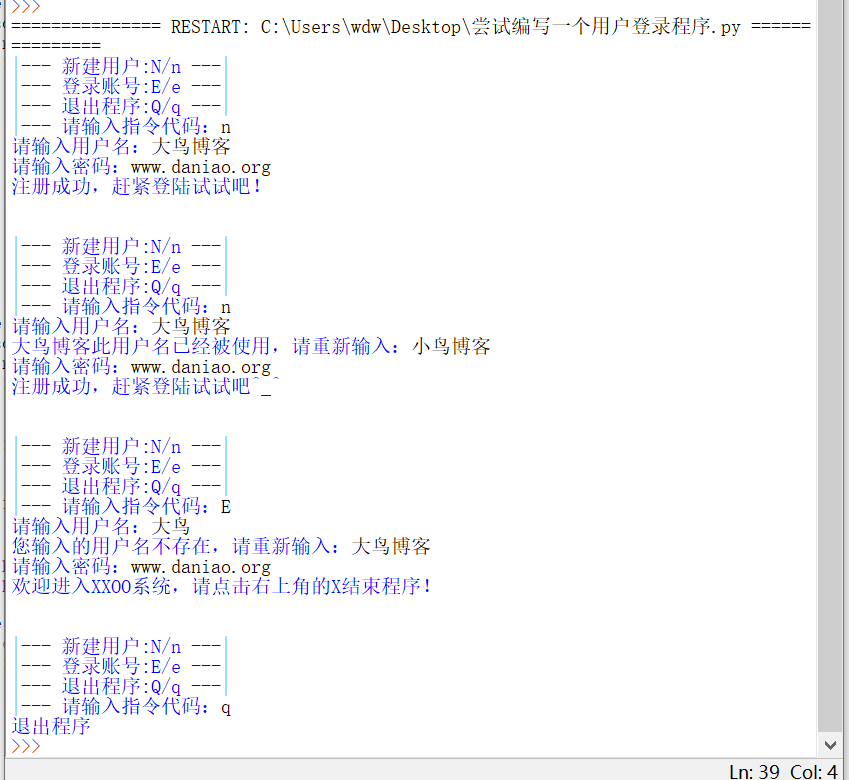# Python 登录系统练习题的设计与改进 – 感觉对函数调用可能有误解

2019年6月28日17:01:0575,823 1857字阅读6分11秒```def log():
print('|--- 新建用户:N/n ---|')
print('|--- 登录账号:E/e ---|')
print('|--- 退出程序:Q/q ---|')
code = input('|--- 请输入指令代码：')
return code

code = log()
if code == 'N' or code == 'n':
name = input('请输入用户名：')
print('注册成功，赶紧登陆试试吧！')
print('\n')

code = log()
if code == 'N' or code == 'n':
name = input('请输入用户名：')
print('%s此用户名已经被使用，请重新输入：' % name ,end='')
name = input()
print('注册成功，赶紧登陆试试吧^_^')
print('\n')
else:
print('用户名不存在，请新建用户')
print('\n')

code = log()
if code == 'E' or code == 'e':
name = input('请输入用户名：')
print('您输入的用户名不存在，请重新输入：',end = '')
name = input()
print('请输入密码：',end = '')
break
print('欢迎进入XXOO系统，请点击右上角的X结束程序！')
print('\n')

code = log()
while 1:
if code == 'Q' or code == 'q':
print('退出程序')
break
```

```user_data = {}

def new_user():
prompt = '请输入用户名：'
while True:
name = input(prompt)
if name in user_data:
prompt = '此用户名已经被使用，请重新输入：'
continue
else:
break

passwd = input('请输入密码：')
user_data[name] = passwd
print('注册成功，赶紧试试登录吧^_^')

def old_user():
prompt = '请输入用户名：'
while True:
name = input(prompt)
if name not in user_data:
prompt = '您输入的用户名不存在，请重新输入：'
continue
else:
break

passwd = input('请输入密码：')
pwd = user_data.get(name)
if passwd == pwd:
print('欢迎进入XXOO系统，请点右上角的X结束程序！')
else:
print('密码错误！')

prompt = '''
|--- 新建用户：N/n ---|
|--- 登录账号：E/e ---|
|--- 推出程序：Q/q ---|
|--- 请输入指令代码：'''

while True:
chosen = False
while not chosen:
choice = input(prompt)
if choice not in 'NnEeQq':
print('您输入的指令代码错误，请重新输入：')
else:
chosen = True

if choice == 'q' or choice == 'Q':
break
if choice == 'n' or choice == 'N':
new_user()
if choice == 'e' or choice == 'E':
old_user()•心灵博客 5

你写的代码也是高大上！我都不怎么会。早先学过点python，忘光了。

•大鸟

@ 心灵博客 空了还可以在把书本捡起来学学！

•WRZ 0

谢谢教程，是准备自学python!

•心灵博客 5

@ WRZ 学go吧

•大鸟

@ WRZ 自学就是找虐的过程啊。但是方法很重要！

•奶爸de笔记 7

厉害呀，你又学这个去了。

•云点SEO 6

python以前略懂皮毛，现在毛都忘了